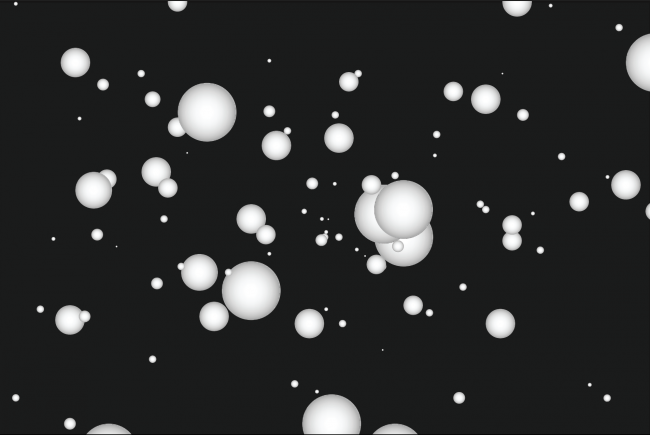#### Howdy, Stranger!

We are about to switch to a new forum software. Until then we have removed the registration on this forum.

# How to increase our camera in processing?

edited June 2016

I'm trying to keep the balls in the screen but they they keep disapearinvg in the z axis. I dont know if i'm doing anything wrong with the forces, or if there's any way i could manipulate the camera caracters, to make it bigger. The Z axis seems to be very "short".

Here's what i've got so far:

``````Ball[] balls;
int numBalls = 0;
Ball b;
boolean isPressed = false;

void setup() {
size (800, 800, P3D);
smooth();
//camera(width/2.0, height/2.0, (height/2.0) / tan(PI*30.0 / 180.0), width/2.0, height/2.0, 0, 0, 1, 0);
background (0);
balls = new Ball;
}

void draw (){
noStroke();
fill (0);
rect(0, 0, width, height);
if (mousePressed){

//println(isPressed, numBalls);
if(isPressed == false && numBalls < 300){
println("cria bola");
b = new Ball(mouseX, mouseY, 200.0);
balls[numBalls] = b;
numBalls++;
isPressed = true;
}

}else{
isPressed = false;
}
println("NUMBALLS:", numBalls);
for( int i = 0; i<numBalls; i++){
//println(">", i,  balls[i]);
balls[i].update();
}

for( int i = 0; i<numBalls; i++){
balls[i].draw();
}

}

public class Ball {

PVector location;
PVector velocity;
PVector acceleration;
PVector gravity;
PVector lance;
float friction ;
int depth;

Ball(float x, float y, float z) {

location = new PVector (x, y, z);
lance = new PVector (0, -20, -15);
velocity = new PVector(0, 0, 0);
//println("created:", location.x, location.y);
}
void update(){
//println(location.x,location.y,location.z, this);
lance = new PVector(0, 0, 0);

if ((location.z < 10)) {
friction = 0.76;
gravity = new PVector(0, 0, 0);
} else {
friction = 1.0;
gravity = new PVector(0, 0.98, 0);
}

depth = 800;
smooth();
//gravity = new PVector(0, 0.98, 0);
//location = new PVector(width/2, height/2, -3000);
//velocity = new PVector(0, 10, -20);
//lance = new PVector(0, 0, 0);
//friction = 1.0; //0.98;

velocity.mult(friction);

}
void draw(){
pushMatrix();
//println("draw", this);
noStroke();
fill(255);
lights();
translate(location.x, location.y, location.z);
sphere(35);
popMatrix();
}
}
``````

(I' want to make something like this picture, by making random forces in the lances. )

Thank you so muchTagged: